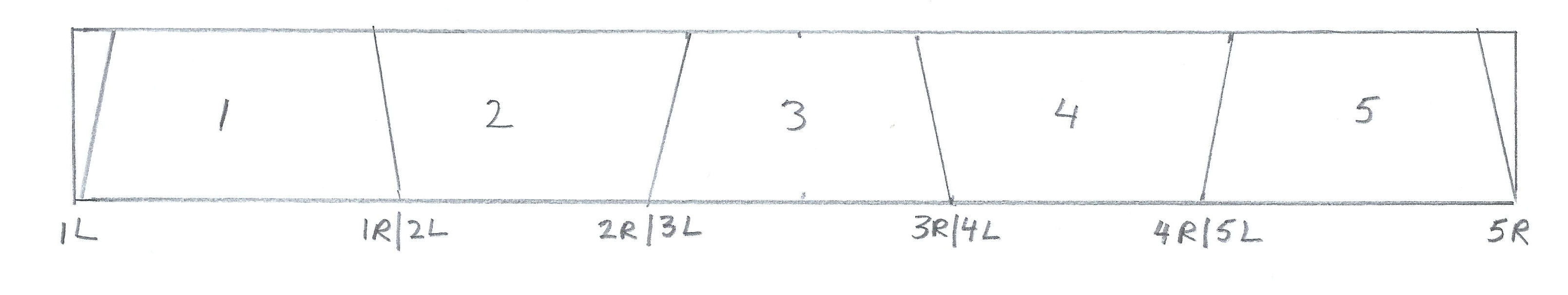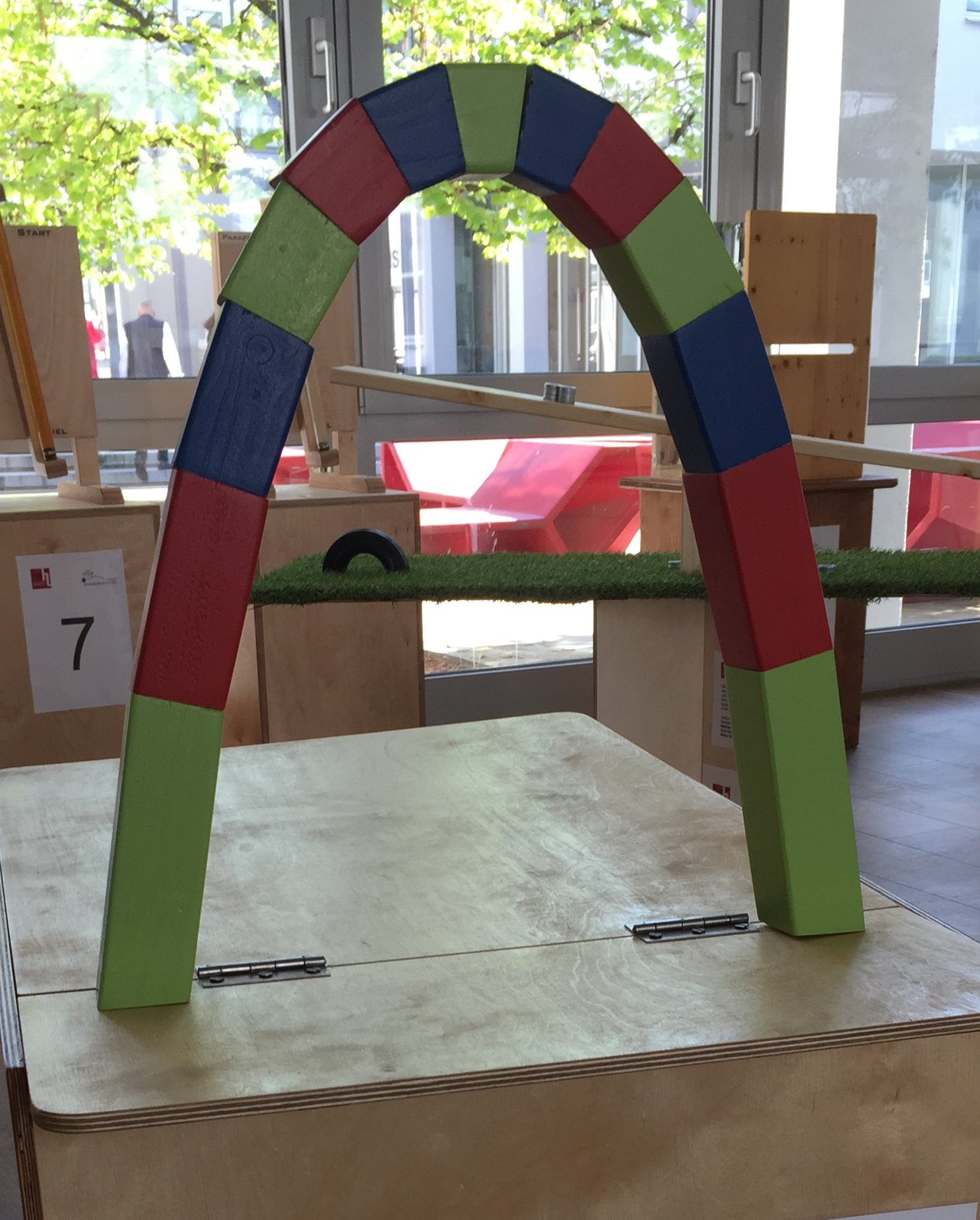Making a Catenary Arch out of Wood
y = a cosh(x/a) (inverted)

Number of Blocks: Input shape parameter a:
 Block End x(cuts) y(cuts) Block Length Block Angle Cut Angle 1L Deg Deg 1R|2L Deg Deg 2R|3L Deg Deg 3R|4L Deg Deg 4R|5L Deg Deg 5R|6L Deg Deg 6R|7L Deg Deg 7R|8L Deg Deg 8R|9L Deg Deg 9R|10L Deg Deg 10R|11L Deg Deg 11R|12L Deg Deg 12R|13L Deg Deg 13R|14L Deg Deg 14R|15L Deg Deg 15R Deg

Block End: The block end columns are lables of the right and left end of each block. For example, 1R|2L means the right end of block one and the left end of block 2. 2R|3L is the other end, right end, of block 2 and the left end of block 3.
x(cuts),y(cuts): These are the x and y coordinates of the block ends. Note: The highest point of the arch is at x=0. So, it is best to have the x values be symmetric about x=0.
Block Length: This is the length of the block, from the midpoint of the left end to the midpoint of the right end.
Block Angle: This is the angle that the block makes with the horizontal. A negative value means that the right end is lower than the left end.
Cut Angle: This is the angle that the wood needs to be cut. A perpendicular cut equals zero degrees. See the diagram below.

Type in the number of blocks, 1-15, then click "enter". Next, type in the x(cut) values you want to use. Then input the value for "a" and click "calculate". The block lengths and cut angles are displayed along with a graph of the catenary. The green dots in the graph are the ends of the blocks, and follow the catenary shape y=a cosh(x/a) (inverted). The blue lines are the centerlines of the blocks.

Below, the diagram on the left shows how one can cut a length of wood to make a 5 block catenary. If you draw the cut lines before cutting, remember that the saw blade has a thickness. The picture on the right is the catenary we made out of 13 blocks. Our x(cut) values were: -19.5, -16.5, -13.5, -10.5, -7.5, -4.5, -1.5, 1.5, 4.5, 7.5, 10.5, 13.5, 16.5, and 19.5 (cm). Our value for a is 7.5.